### LIMITS OF FUNCTIONS USING THE SQUEEZE PRINCIPLE

The following problems involve the algebraic computation of limits using the Squeeze Principle, which is given below.

SQUEEZE PRINCIPLE : Assume that functions f , g , and h satisfy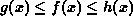and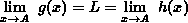.

Then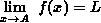.

(NOTE : The quantity A may be a finite number,, or. The quantitiy L may be a finite number,, or.)

The Squeeze Principle is used on limit problems where the usual algebraic methods (factoring, conjugation, algebraic manipulation, etc.) are not effective. However, it requires that you be able to ``squeeze'' your problem in between two other ``simpler'' functions whose limits are easily computable and equal. The use of the Squeeze Principle requires accurate analysis, deft algebra skills, and careful use of inequalities.

• PROBLEM 1 : Compute.

• PROBLEM 2 : Compute.

• PROBLEM 3 : Compute.

• PROBLEM 4 : Compute.

• PROBLEM 5 : Compute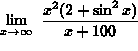.

• PROBLEM 6 : Compute.

• PROBLEM 7 : Compute.

• PROBLEM 8 : Assume thatexists and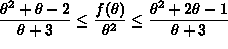. Find.

• PROBLEM 9 : Consider a circle of radius 1 centered at the origin and an angle ofradians,, in the given diagram.a.) By considering the areas of right triangle OAD, sector OAC, and right triangle OBC, conclude that.

b.) Use part a.) and the Squeeze Principle to show that• PROBLEM 10 : Assume that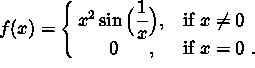Show that f is continuous at x=0 .